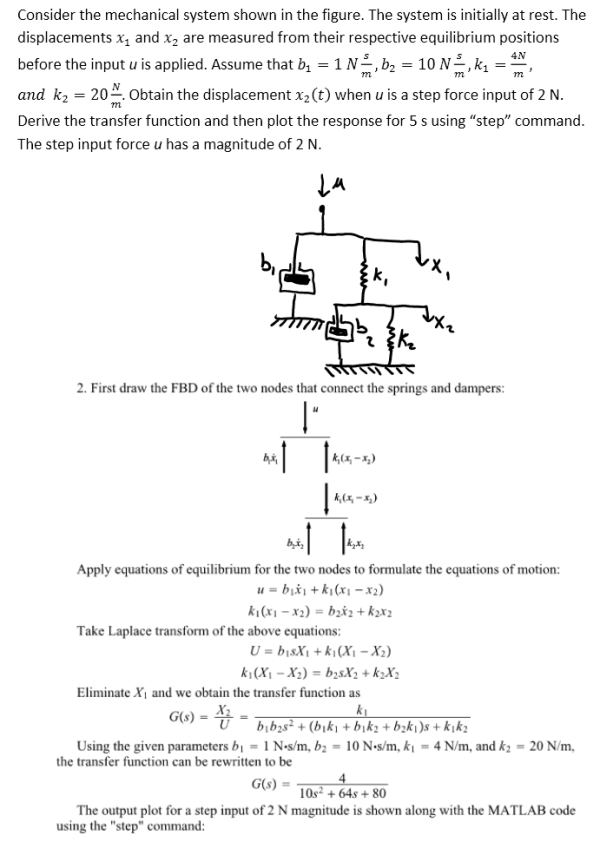Consider the mechanical system shown in the figure. The system is initially at rest. The displacements x1 and x2 are measured from their respective equilibrium positions before the input u is applied. Assume that b1=Ns/m, b2=10Ns/m, k1=4N/m, and k2=20N/m. Obtain the displacement x2(t) when u is a step force input of 2 N. Derive the transfer function and then plot the response for 5 s using step command. The step input force u has a magnitude of 2 N.Consider the mechanical system shown in the figure. The system is initially at rest. The displacements x1 and x2 are measured from their respective equilibrium positions before the input u is applied. Assume that b1=Ns/m, b2=10Ns/m, k1=4N/m, and k2=20N/m. Obtain the displacement x2(t) when u is a step force input of 2 N. Derive the transfer function and then plot the response for 5 s using step command. The step input force u has a magnitude of 2 N.

System Dynamics Page 2 dynamics dynamics dynamics dynamics dynamics dynamics dynamics System dynamics Page 3